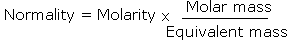What is the relation between normality and molarity of a solution?

#1

What is the relation between normality and molarity of a solution ?

#2

Normality and Molarity are related as:For acids

Normality = Molarity x Basicity

where basicity is the number of H+ ions a molecule of an acid can give

For bases

Normality = Molarity x Acidity

where acidity is the number of OH- ions a molecule of a base can give.